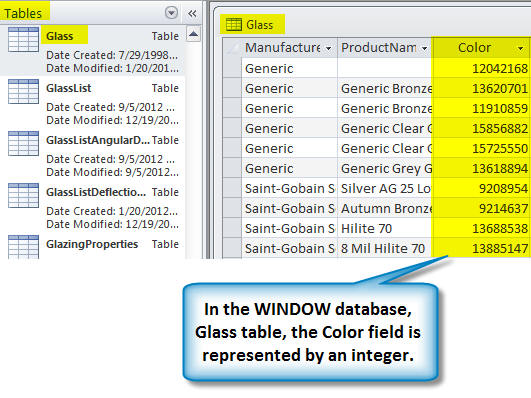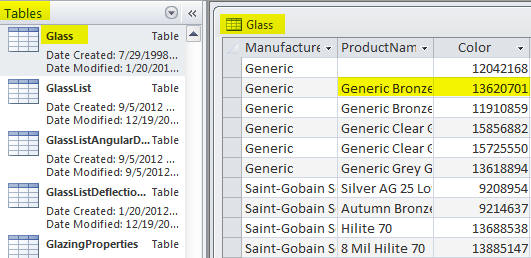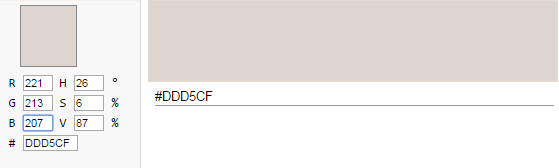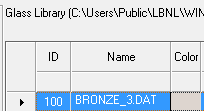# Glass Color in WINDOW

Last Updated:
Friday, September 1, 2017

## Converting to the RGB Model

In the WINDOW database, the color value in the Glass table is represented as an integer.In order to convert that integer to the RGB color model, do the following:

• convert the integer to hex
• then convert each pair of hex values to an integer
• those 3 pairs are the RGB values in the order BLUE, GREEN, RED (not red, green blue !)

## Example

### NFRC ID 100 – BronzeInteger value in DB

• 13620701

Convert to hex:

• cfd5dd

Convert the three paired hex values back to integers:

• cf : 207
• d5: 213
• dd = 221

The color value in database is bbggrr (BLUE/GREEN/RED).

So for NFRC ID 100

• cf = blue = 207
• d5 = green = 213
• dd = red = 221

Using an RGB color display tool, using those integers for RGB, you get